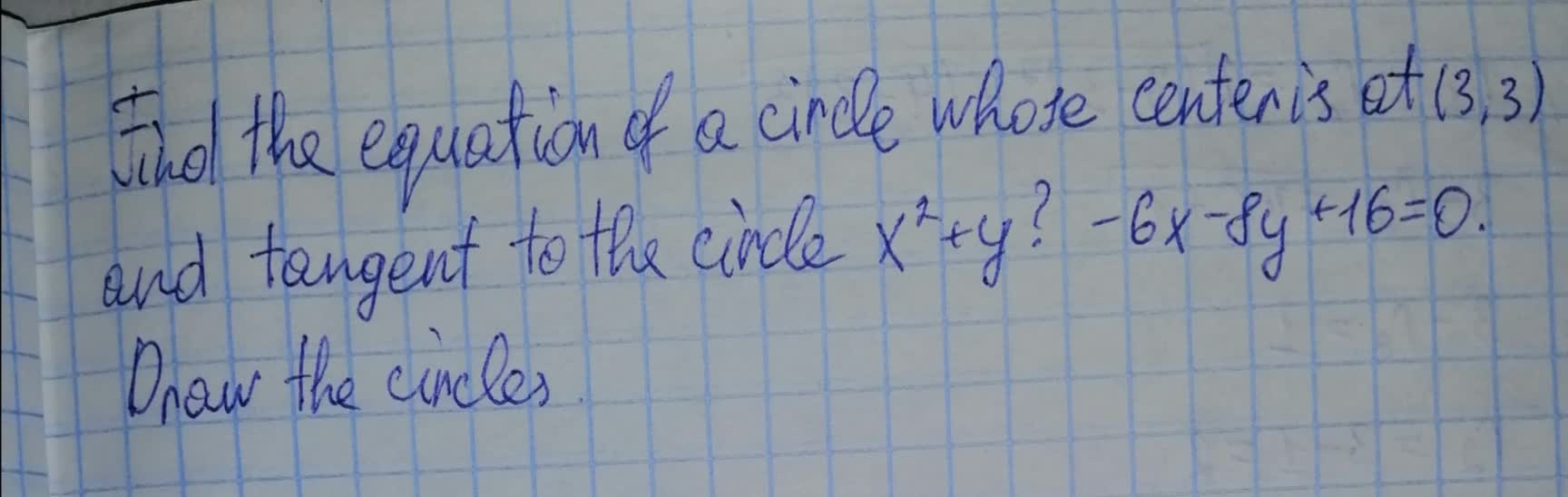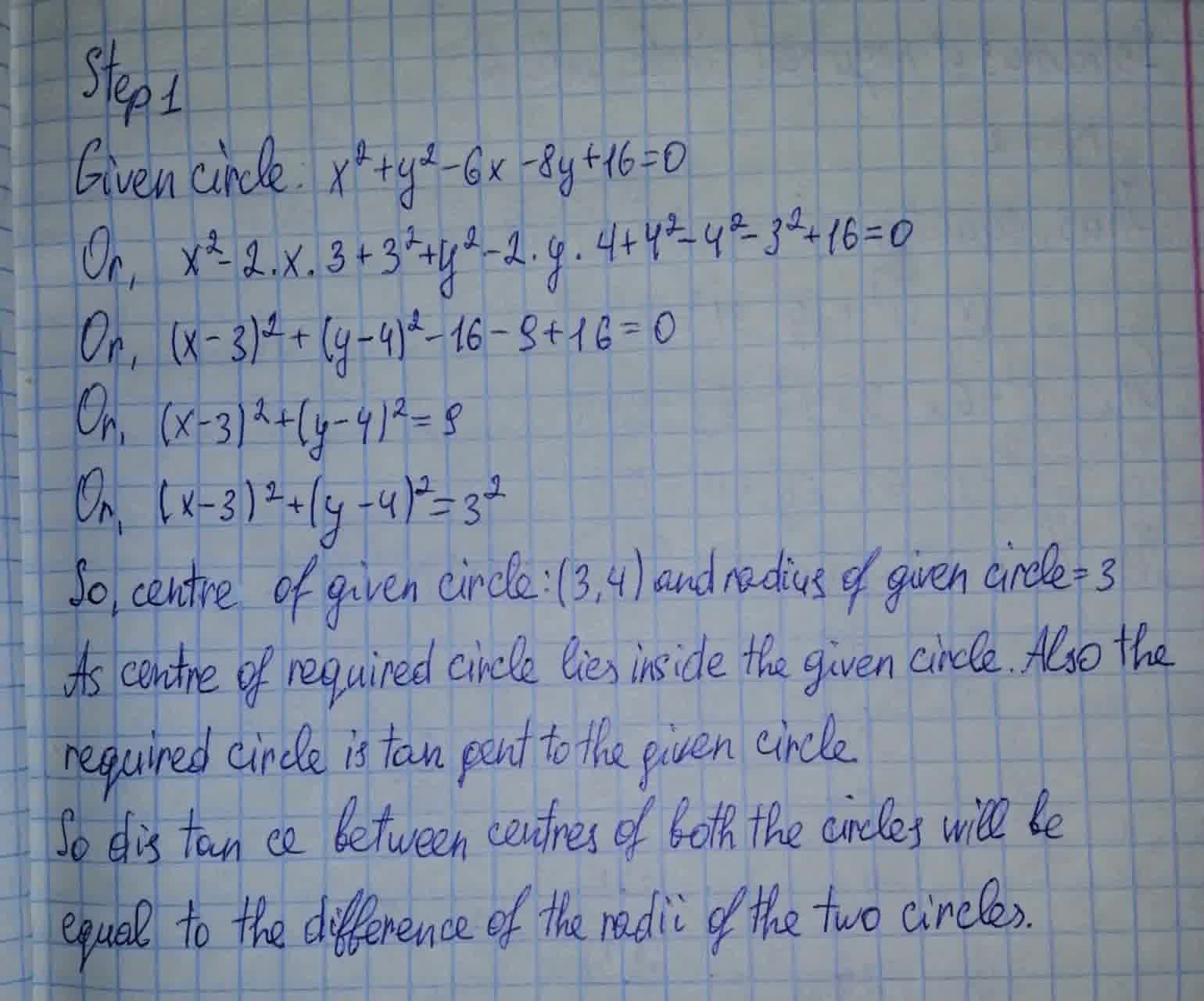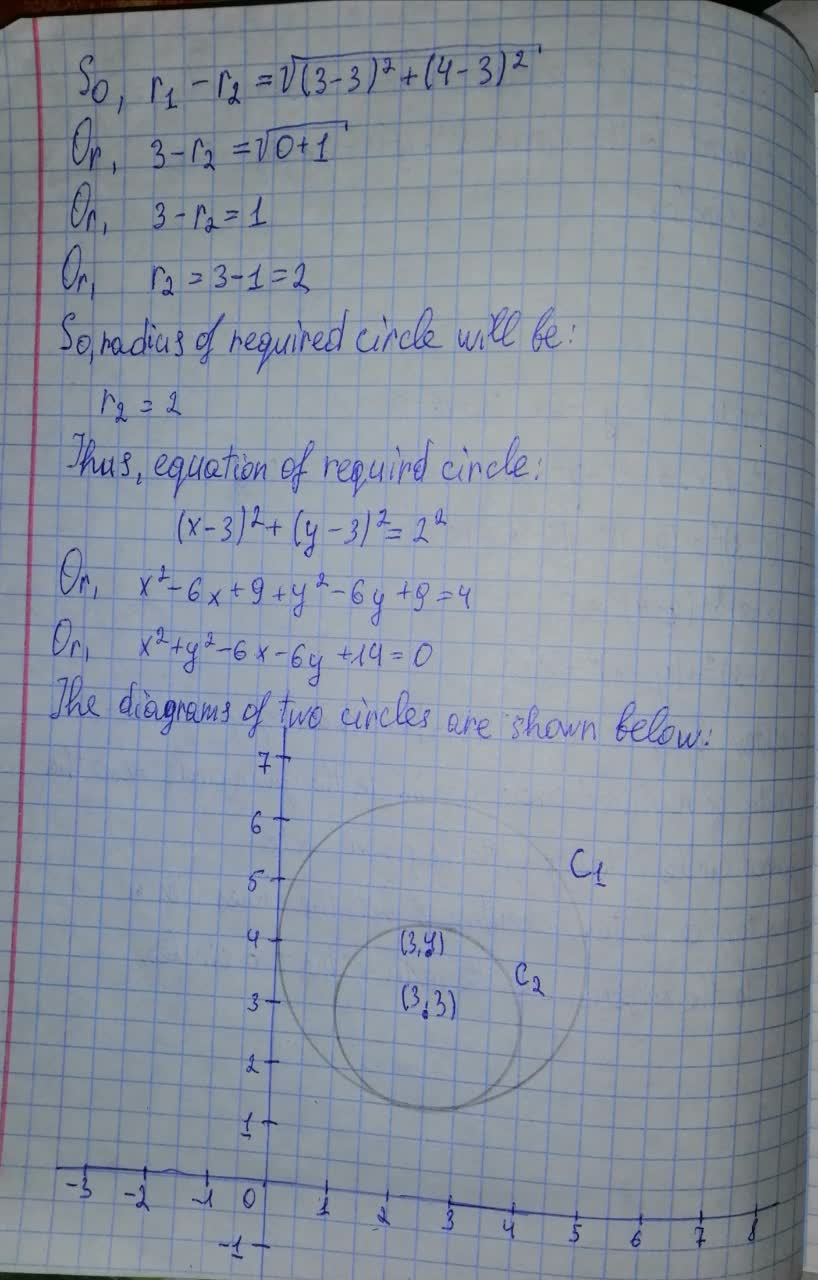Question# Find the equation of a circle whose center is at (3, 3) and tangent to the circle x^2+ y^2 - 6x - 8y + 16 = 0. Draw the circles.

Circles
ANSWEREDFind the equation of a circle whose center is at (3, 3) and tangent to the circle $$x^2+ y^2 - 6x - 8y + 16 = 0$$. Draw the circles.